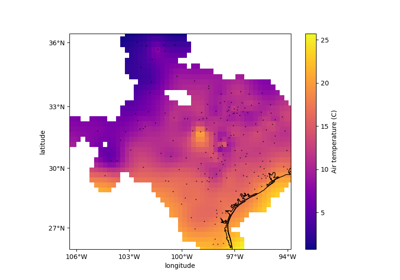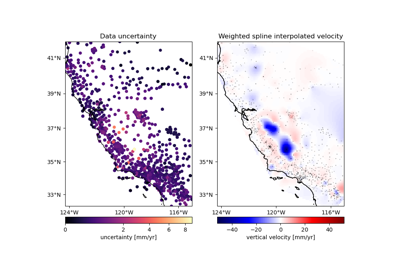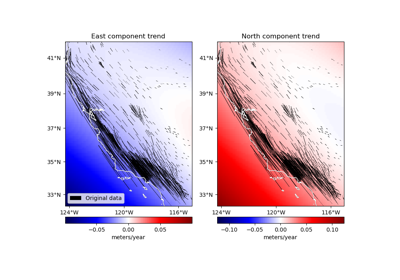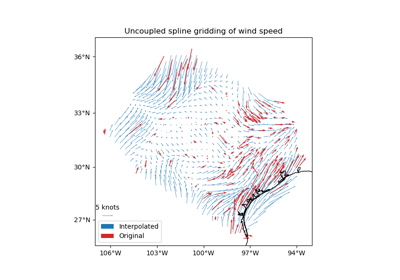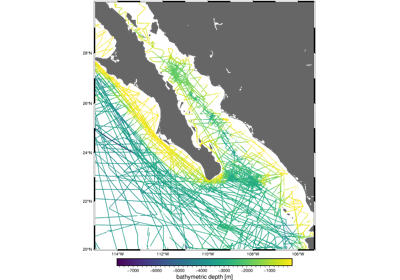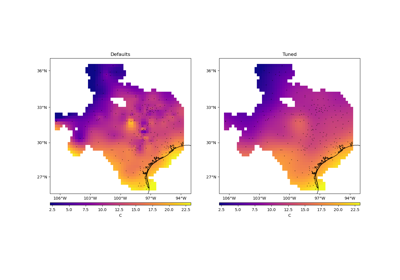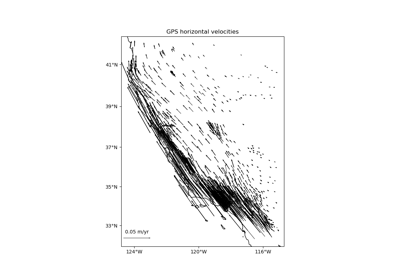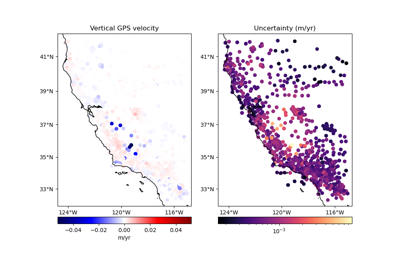# verde.Chain¶

class verde.Chain(steps)[source]

Chain filtering operations to fit on each subsequent output.

The `filter` method of each element of the set is called with the outputs of the previous one. For gridders and trend estimators this means that each element fits the residuals (input data minus predicted data) of the previous one.

When predicting data, the predictions of each step in the chain are added together. Steps that don’t implement a `predict` method are ignored.

This provides a convenient way to chaining operations like trend estimation to a given gridder.

Parameters

steps (list) – A list of `('name', step)` pairs where `step` is any verde class that implements a `filter(coordinates, data, weights)` method (including `Chain` itself).

Variables
• region_ (tuple) – The boundaries (`[W, E, S, N]`) of the data used to fit the chain. Used as the default region for the `grid` and `scatter` methods.

• named_steps (dict) – A dictionary version of steps where the `'name'` strings are keys and the estimator/gridder/processor objects are the values.

`verde.Vector`

Fit an estimator to each component of vector data

Methods Summary

 `Chain.filter`(coordinates, data[, weights]) Filter the data through the gridder and produce residuals. `Chain.fit`(coordinates, data[, weights]) Fit the chained estimators to the given data. `Chain.get_params`([deep]) Get parameters for this estimator. `Chain.grid`([region, shape, spacing, dims, ...]) Interpolate the data onto a regular grid. `Chain.predict`(coordinates) Evaluates the data predicted by the chain on the given set of points. `Chain.profile`(point1, point2, size[, dims, ...]) Interpolate data along a profile between two points. `Chain.scatter`([region, size, random_state, ...]) Interpolate values onto a random scatter of points. `Chain.score`(coordinates, data[, weights]) Score the gridder predictions against the given data. `Chain.set_params`(**params) Set the parameters of this estimator.

Chain.filter(coordinates, data, weights=None)

Filter the data through the gridder and produce residuals.

Calls `fit` on the data, evaluates the residuals (data - predicted data), and returns the coordinates, residuals, and weights.

No very useful by itself but this interface makes gridders compatible with other processing operations and is used by `verde.Chain` to join them together (for example, so you can fit a spline on the residuals of a trend).

Parameters
• coordinates (tuple of arrays) – Arrays with the coordinates of each data point. Should be in the following order: (easting, northing, vertical, …). For the specific definition of coordinate systems and what these names mean, see the class docstring.

• data (array or tuple of arrays) – The data values of each data point. If the data has more than one component, data must be a tuple of arrays (one for each component).

• weights (None or array or tuple of arrays) – If not None, then the weights assigned to each data point. If more than one data component is provided, you must provide a weights array for each data component (if not None).

Returns

coordinates, residuals, weights – The coordinates and weights are same as the input. Residuals are the input data minus the predicted data.

Chain.fit(coordinates, data, weights=None)[source]

Fit the chained estimators to the given data.

Each estimator in the chain is fitted to the residuals of the previous estimator. The coordinates are preserved. Only the data values are changed.

The data region is captured and used as default for the `grid` and `scatter` methods.

All input arrays must have the same shape.

Parameters
• coordinates (tuple of arrays) – Arrays with the coordinates of each data point. Should be in the following order: (easting, northing, vertical, …).

• data (array or tuple of arrays) – the data values of each data point. if the data has more than one component, data must be a tuple of arrays (one for each component).

• weights (none or array or tuple of arrays) – if not none, then the weights assigned to each data point. if more than one data component is provided, you must provide a weights array for each data component (if not none).

Returns

self – Returns this estimator instance for chaining operations.

Chain.get_params(deep=True)

Get parameters for this estimator.

Parameters

deep (bool, default=True) – If True, will return the parameters for this estimator and contained subobjects that are estimators.

Returns

params (dict) – Parameter names mapped to their values.

Chain.grid(region=None, shape=None, spacing=None, dims=None, data_names=None, projection=None, coordinates=None, **kwargs)

Interpolate the data onto a regular grid.

The grid can be specified by two methods:

Use the dims and data_names arguments to set custom names for the dimensions and the data field(s) in the output `xarray.Dataset`. Default names will be provided if none are given.

Parameters
• region (list = [W, E, S, N]) – The west, east, south, and north boundaries of a given region. Use only if `coordinates` is None.

• shape (tuple = (n_north, n_east) or None) – The number of points in the South-North and West-East directions, respectively. Use only if `coordinates` is None.

• spacing (tuple = (s_north, s_east) or None) – The grid spacing in the South-North and West-East directions, respectively. Use only if `coordinates` is None.

• dims (list or None) – The names of the northing and easting data dimensions, respectively, in the output grid. Default is determined from the `dims` attribute of the class. Must be defined in the following order: northing dimension, easting dimension. NOTE: This is an exception to the “easting” then “northing” pattern but is required for compatibility with xarray.

• data_names (str, list or None) – The name(s) of the data variables in the output grid. Defaults to `'scalars'` for scalar data, `['east_component', 'north_component']` for 2D vector data, and `['east_component', 'north_component', 'vertical_component']` for 3D vector data.

• projection (callable or None) – If not None, then should be a callable object `projection(easting, northing) -> (proj_easting, proj_northing)` that takes in easting and northing coordinate arrays and returns projected northing and easting coordinate arrays. This function will be used to project the generated grid coordinates before passing them into `predict`. For example, you can use this to generate a geographic grid from a Cartesian gridder.

• coordinates (tuple of arrays) – Tuple of arrays containing the coordinates of the grid in the following order: (easting, northing, vertical, …). The easting and northing arrays could be 1d or 2d arrays, if they are 2d they must be part of a meshgrid. If coordinates are passed, `region`, `shape`, and `spacing` are ignored.

Returns

grid (xarray.Dataset) – The interpolated grid. Metadata about the interpolator is written to the `attrs` attribute.

`verde.grid_coordinates`

Generate the coordinate values for the grid.

Chain.predict(coordinates)[source]

Evaluates the data predicted by the chain on the given set of points.

Predictions from each step in the chain are added together. Any step that doesn’t implement a `predict` method is ignored.

Requires a fitted gridder (see `fit`).

Parameters

coordinates (tuple of arrays) – Arrays with the coordinates of each data point. Should be in the following order: (easting, northing, vertical, …).

Returns

data (array) – The data values predicted on the given points.

Chain.profile(point1, point2, size, dims=None, data_names=None, projection=None, **kwargs)

Interpolate data along a profile between two points.

Generates the profile along a straight line assuming Cartesian distances. Point coordinates are generated by `verde.profile_coordinates`. Other arguments for this function can be passed as extra keyword arguments (`kwargs`) to this method.

Use the dims and data_names arguments to set custom names for the dimensions and the data field(s) in the output `pandas.DataFrame`. Default names are provided.

Includes the calculated Cartesian distance from point1 for each data point in the profile.

To specify point1 and point2 in a coordinate system that would require projection to Cartesian (geographic longitude and latitude, for example), use the `projection` argument. With this option, the input points will be projected using the given projection function prior to computations. The generated Cartesian profile coordinates will be projected back to the original coordinate system. Note that the profile points are evenly spaced in projected coordinates, not the original system (e.g., geographic).

Warning

The profile calculation method with a projection has changed in Verde 1.4.0. Previous versions generated coordinates (assuming they were Cartesian) and projected them afterwards. This led to “distances” being incorrectly handled and returned in unprojected coordinates. For example, if `projection` is from geographic to Mercator, the distances would be “angles” (incorrectly calculated as if they were Cartesian). After 1.4.0, point1 and point2 are projected prior to generating coordinates for the profile, guaranteeing that distances are properly handled in a Cartesian system. With this change, the profile points are now evenly spaced in projected coordinates and the distances are returned in projected coordinates as well.

Parameters
• point1 (tuple) – The easting and northing coordinates, respectively, of the first point.

• point2 (tuple) – The easting and northing coordinates, respectively, of the second point.

• size (int) – The number of points to generate.

• dims (list or None) – The names of the northing and easting data dimensions, respectively, in the output dataframe. Default is determined from the `dims` attribute of the class. Must be defined in the following order: northing dimension, easting dimension. NOTE: This is an exception to the “easting” then “northing” pattern but is required for compatibility with xarray.

• data_names (str, list or None) – The name(s) of the data variables in the output dataframe. Defaults to `'scalars'` for scalar data, `['east_component', 'north_component']` for 2D vector data, and `['east_component', 'north_component', 'vertical_component']` for 3D vector data.

• projection (callable or None) – If not None, then should be a callable object ```projection(easting, northing, inverse=False) -> (proj_easting, proj_northing)``` that takes in easting and northing coordinate arrays and returns projected northing and easting coordinate arrays. Should also take an optional keyword argument `inverse` (default to False) that if True will calculate the inverse transform instead. This function will be used to project the profile end points before generating coordinates and passing them into `predict`. It will also be used to undo the projection of the coordinates before returning the results.

Returns

table (pandas.DataFrame) – The interpolated values along the profile.

Chain.scatter(region=None, size=300, random_state=0, dims=None, data_names=None, projection=None, **kwargs)

Interpolate values onto a random scatter of points.

Point coordinates are generated by `verde.scatter_points`. Other arguments for this function can be passed as extra keyword arguments (`kwargs`) to this method.

If the interpolator collected the input data region, then it will be used if `region=None`. Otherwise, you must specify the grid region.

Use the dims and data_names arguments to set custom names for the dimensions and the data field(s) in the output `pandas.DataFrame`. Default names are provided.

Parameters
• region (list = [W, E, S, N]) – The west, east, south, and north boundaries of a given region.

• size (int) – The number of points to generate.

• random_state (numpy.random.RandomState or an int seed) – A random number generator used to define the state of the random permutations. Use a fixed seed to make sure computations are reproducible. Use `None` to choose a seed automatically (resulting in different numbers with each run).

• dims (list or None) – The names of the northing and easting data dimensions, respectively, in the output dataframe. Default is determined from the `dims` attribute of the class. Must be defined in the following order: northing dimension, easting dimension. NOTE: This is an exception to the “easting” then “northing” pattern but is required for compatibility with xarray.

• data_names (str, list or None) – The name(s) of the data variables in the output dataframe. Defaults to `'scalars'` for scalar data, `['east_component', 'north_component']` for 2D vector data, and `['east_component', 'north_component', 'vertical_component']` for 3D vector data.

• projection (callable or None) – If not None, then should be a callable object `projection(easting, northing) -> (proj_easting, proj_northing)` that takes in easting and northing coordinate arrays and returns projected northing and easting coordinate arrays. This function will be used to project the generated scatter coordinates before passing them into `predict`. For example, you can use this to generate a geographic scatter from a Cartesian gridder.

Returns

table (pandas.DataFrame) – The interpolated values on a random set of points.

Chain.score(coordinates, data, weights=None)

Score the gridder predictions against the given data.

Calculates the R^2 coefficient of determination of between the predicted values and the given data values. A maximum score of 1 means a perfect fit. The score can be negative.

Warning

The default scoring will change from R² to negative root mean squared error (RMSE) in Verde 2.0.0. This may change model selection results slightly. The negative version will be used to maintain the behaviour of larger scores being better, which is more compatible with current model selection code.

If the data has more than 1 component, the scores of each component will be averaged.

Parameters
• coordinates (tuple of arrays) – Arrays with the coordinates of each data point. Should be in the following order: (easting, northing, vertical, …). For the specific definition of coordinate systems and what these names mean, see the class docstring.

• data (array or tuple of arrays) – The data values of each data point. If the data has more than one component, data must be a tuple of arrays (one for each component).

• weights (None or array or tuple of arrays) – If not None, then the weights assigned to each data point. If more than one data component is provided, you must provide a weights array for each data component (if not None).

Returns

score (float) – The R^2 score

Chain.set_params(**params)

Set the parameters of this estimator.

The method works on simple estimators as well as on nested objects (such as `Pipeline`). The latter have parameters of the form `<component>__<parameter>` so that it’s possible to update each component of a nested object.

Parameters

**params (dict) – Estimator parameters.

Returns

self (estimator instance) – Estimator instance.

## Examples using `verde.Chain`¶